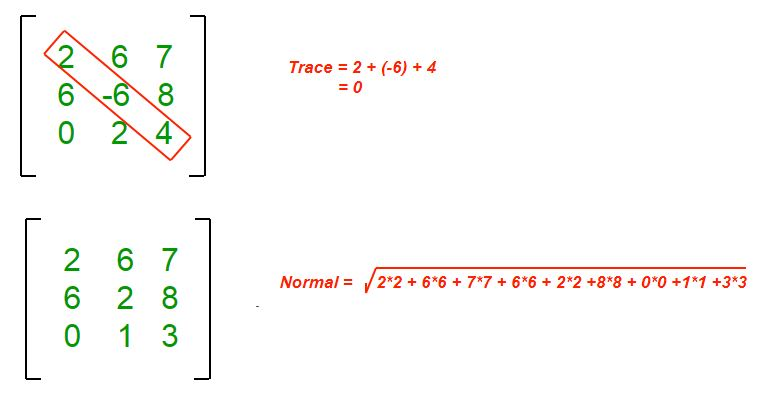# Java Program to Find the Normal and Trace of a Matrix

• Last Updated : 23 May, 2022

For a given 2D matrix, the purpose is to find the Trace and Normal of the matrix.

Normal of a matrix is defined as the square root of the sum of squares of all the elements of the matrix.

Trace of a given square matrix is defined as the sum of all the elements in the diagonal.

Examples :

```Input : matrix[][] = {{1, 4, 4},
{2, 3, 7},
{0, 5, 1}};
Output : Normal = 11
Trace  = 5
Explanation :
Normal = sqrt(1*1+ 4*4 + 4*4 + 2*2 +
3*3 + 7*7 + 0*0 + 5*5 + 1*1)
= 11
Trace  = 1+3+1 = 5

Input :matrix[][] = {{8, 9, 11},
{0, 1, 15},
{4, 10, -7}};
Output : Normal = 25
Trace  = 2
Explanation :
Normal = sqrt(8*8 +9*9 + 11*11 + 0*0 + 1*1 +
15*15 + 4*4 + 10*10 + -7*-7) = 25
Trace  = (8+1-7) = 2```Example:

## Java

 `// Java program to find the trace``// and normal of the given matrix` `import` `java.io.*;` `class` `geeksforgeeks {` `    ``// Dimension of the given matrix``    ``static` `int` `max = ``50``;` `    ``// Finds Normal of the given``    ``// matrix of size N x N``    ``static` `int` `Normal(``int` `matrix[][], ``int` `N)``    ``{``        ``// Initializing sum``        ``int` `s = ``0``;``        ``for` `(``int` `j = ``0``; j < N; j++)``            ``for` `(``int` `k = ``0``; k < N; k++)``                ``s += matrix[j][k] * matrix[j][k];``        ``return` `(``int``)Math.sqrt(s);``    ``}` `    ``// Finds trace of the given``    ``// matrix of size N x N``    ``static` `int` `Trace(``int` `matrix[][], ``int` `N)``    ``{``        ``int` `s = ``0``;``        ``for` `(``int` `j = ``0``; j < N; j++)``            ``s += matrix[j][j];``        ``return` `s;``    ``}` `    ``// The Driver code``    ``public` `static` `void` `main(String[] args)``    ``{` `        ``int` `matrix[][] = {``            ``{ ``2``, ``3``, ``5``, ``6``, ``7` `},      { ``8``, ``9``, ``10``, ``11``, ``12` `},``            ``{ ``13``, ``14``, ``15``, ``16``, ``17` `}, { ``18``, ``1``, ``3``, ``0``, ``6` `},``            ``{ ``7``, ``8``, ``11``, ``8``, ``11` `},``        ``};` `        ``System.out.println(``"Trace of the Matrix is: "``                           ``+ Trace(matrix, ``5``));``        ``System.out.println(``"Normal of the Matrix is: "``                           ``+ Normal(matrix, ``5``));``    ``}``}`

Output

```Trace of the Matrix is: 37
Normal of the Matrix is: 50```

Time Complexity: O(N*N), as we are using nested loops for traversing the matrix.

Auxiliary Space: O(1), as we are not using any extra space.

My Personal Notes arrow_drop_up